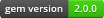# shoulda-contextOfficial Documentation

Shoulda's contexts make it easy to write understandable and maintainable tests for Test::Unit. It's fully compatible with your existing tests in Test::Unit, and requires no retooling to use.

Refer to the shoulda gem if you want to know more about using shoulda with Rails or RSpec.

## Contexts

Instead of writing Ruby methods with `lots_of_underscores`, shoulda-context adds context, setup, and should blocks...

``````require "test/unit"

class CalculatorTest < Test::Unit::TestCase
context "a calculator" do
setup do
@calculator = Calculator.new
end

should "add two numbers for the sum" do
assert_equal 4, @calculator.sum(2, 2)
end

should "multiply two numbers for the product" do
assert_equal 10, @calculator.product(2, 5)
end
end
end
``````

... which combine to produce the following test methods:

``````"test: a calculator should add two numbers for the sum."
"test: a calculator should multiply two numbers for the product."
``````

When running a single test method via (example from a Rails context):

``````ruby -I"lib:test" path_to_test_file -n name_of_the_test_method
``````

you should include a trailing space(!), e.g.,

``````ruby -I"lib:test" path_to_test_file -n "test: a calculator should add two numbers for the sum. "
``````

## Assertions

It also has two additional Test::Unit assertions for working with Ruby's Array:

``````assert_same_elements([:a, :b, :c], [:c, :a, :b])
assert_contains(['a', '1'], /\d/)
assert_contains(['a', '1'], 'a')
``````

## Credits

Shoulda is maintained and funded by thoughtbot. shoulda-context is maintained by Travis Jeffery. Thank you to all the contributors.